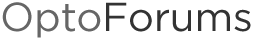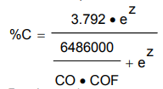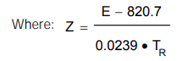# Complex math in a function node

I am hoping for some guidance on how to perform (somewhat) complex math in Node-RED. My current flow that grabs some parameters from SQL and puts them into my strategy looks like this:

I need to perform some complex math using these two values. Specifically, if H4 and H5 are the values being retrieved from SQL, the formula that I want to use to produce a new value is:

(3.792*(2.71828^((H5-820.7)/(0.0239H4))))
/
(1621.5+(2.71828^((H5-820.7)/(0.0239
H4))))

I believe (acc. to this post) that I need to create a function node that contains something like this:

``````#include <cmath>

return msg;
``````

Based on the above, I can figure out how to write the formula in msg.payload, but where do I put a function node in my flow? It seems I would need to put it after the two groov write nodes.

Thank you!

When it comes to the math formula I suggest using `Math.pow(x,y)` to calculate `x^y`. This method works great in Node-RED and doesn’t rely on any #include statements, which will not work in function nodes as far as I know.

Regarding the flow placement I would try putting a third split from the MSSQL node so that both your PAC Control tags and the Node-RED function are getting the exact same values. It should also help minimize any potential delays:

Thank you. I am now working on building the formula, which means educating myself on the math functions, but I cannot get even the Math.add function to work.

This works:
return msg;

returning 1750 squared, i.e. the following output

3062500

but this does not:

return msg;

returns the following output:

function : (error)

“TypeError: Math.add is not a function”

Maybe Node-red does not support this Math.add function?

Regardless, I eventually stumbled onto defining variables, which were quite easy and will make the calculation easier. For example, this works fine:

var a = 1+3
return msg;

Technically you should be able to do the whole formula in one line, but you’re absolutely right that breaking it down into variables makes long formulas a lot easier to work with. The standard `+ - * /` and `Math.pow()` function should be all you need here.

I may have gotten the H4 and H5 from your formula backwards, but here’s a quick attempt at what it could look like:

``````// create shorter references for message properties

a = (temp-820.7)/(0.0239*time); // part of the numerator
b = (temp-820.7)/(0.0239*time); // part of the denominator

numerator = 3.792 * Math.pow(2.71828, a); // plug in "a"
denominator = 1621.5 + Math.pow(2.71828, b); // plug in "b"